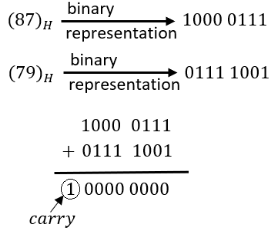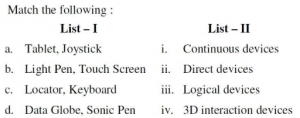## UGC NET CS 2014 Dec - paper-3

 Question 1
A hierarchical memory system that uses cache memory has cache access time of 50 nano seconds, main memory access time of 300 nanoseconds, 75% of memory requests are for read, hit ratio of 0.8 for read access and the write-through scheme is used. What will be the average access time of the system both for read and write requests ?
 A 157.5 n.sec. B 110 n.sec. C 75 n.sec D 82.5 n.sec.
Computer-Organization       Memory-hierarchy
Question 1 Explanation:
Avg. time to read the data = 0.8(50) + 0.2(300+50) = 40 + 70 = 110 ns
Avg. memory access time = (Seq. of data read request * time to read data) + (freq. Of data write request * time to write the data)
= (0.75 × 110) + (0.25 × 300)
⤿Since write through is mentioned in question. So write operation will take place in memory and not in cache.
= 82.5 + 75.0
= 157.5 ns
 Question 2
For switching from a CPU user mode to supervisor mode following type of interrupt is most appropriate
 A Internal interrupts B External interrupts C Software interrupts D None of the above
Computer-Organization       Interrupts
Question 2 Explanation:
Software interrupt is caused either by an exceptional condition in the processor itself, or a special instruction in the instruction set which causes an interrupt when it is executed.
It is used for switching from a CPU user mode to supervisor mode.
Software interrupts is internal interrupt triggered by some software instruction.
There are 8 software interrupts in 8085 microprocessor. They are – RST 0, RST 1, RST 2, RST 3, RST 4, RST 5, RST 6, RST 7.
External interrupt: External interrupts are the interrupts caused by the hardware module. pressing a key on the keyboard or moving the mouse triggers hardware interrupts that cause the processor to read the keystroke or mouse position.
 Question 3
In a dot matrix printer to print a character is 6 m.sec., time to space in between characters is 2 m.sec., and the number of characters in a line are 200. The printing speed of the dot matrix printer in characters per second and the time to print a character line are given by which of the following options ?
 A 125 chars/second and 0.8 seconds B 250 chars/second and 0.6 seconds C 166 chars/second and 0.8 seconds D 250 chars/second and 0.4 seconds E None of the above
Computer-Organization       Graphics
Question 3 Explanation:
If we have 3 characters then the space between two characters will be 2. It means if we have “n” characters in a line then the number of spaces in the line will be “(n-1)”.
So, the time to print a character line = (200*6)+(199*2)
= 1598 ms
= 1598*10-3 second
= 1.59 seconds
Printing speed of the dot matrix printer in characters per second
= 200/ 1.59 character per second
= 125.78 character per second
 Question 4A a-iv, b-i, c-iii, d-ii B a-iii, b-ii, c-i, d-iv C a-ii, b-iii, c-i, d-iv D a-ii, b-iv, c-i, d-iii
Computer-Organization       Flags
Question 4 Explanation:
XCNG: Exchange instruction does not change any flag.
SUB: Subtraction instruction will change all the flags.
STC: Set carry instruction will affect the carry flag only.
DCR: Decrement instruction will decrease the value hence it will effect all flags but not carry flag.
 Question 5
How many times will the following loop be executed ? LXI B, 0007 H LOP : DCX B MOV A, B ORA C JNZ LOP
 A 05 B 07 C 09 D 00
Computer-Organization       Machine-Instructions
Question 5 Explanation:
LXI B, 0007 H // Load register pair B with value (00)H and C with value (07)H
LOP :
DCX B // Decrement register pair BC i.e. decrease C because B is already 0.
MOV A, B // Move value of B to A. It means A is 0 after first iteration of loop LOP.

ORA C // It means perform OR operation between register A and C and store the result into register A. So A = (06)H after first iteration of loop.

So this way LOP will be executed 7 times.
 Question 6
Specify the contents of the accumulator and the status of the S, Z and CY flags when 8085 microprocessor performs addition of 87 H and 79 H.
 A 11, 1, 1, 1 B 10, 0, 1, 0 C 01, 1, 0, 0 D 00, 0, 1, 1
Computer-Organization       Microprocessor
Question 6 Explanation:Since MSB of 8-bit result is zero, it means the number is positive. So Sign flag will be “0”.
Since 8-bit result contain zero only so Zero flag will be “1”.
Since addition operation is resulting into a carry so Carry flag will be “1”.
 Question 7

Location transparency allows :

I. Users to treat the data as if it is done at one location.

II. Programmers to treat the data as if it is at one location.

III. Managers to treat the data as if it is at one location.

Which one of the following is correct ?
 A I, II and III B I and II only C II and III only D II only
Computer-Networks       Security
Question 7 Explanation:
Location Transparency: The name of a file does not reveal any hint of the file's physical storage location i.e. it makes the user, programmers to treat the data as if it is present at one location.
 Question 8

Which of the following is correct ?

I. Two phase locking is an optimistic protocol.

II. Two phase locking is pessimistic protocol

III. Time stamping is an optimistic protocol.

IV. Time stamping is pessimistic protocol.
 A I and III B II and IV C I and IV D II and III
Database-Management-System       Transactions
 Question 9
__________ rules used to limit the volume of log information that has to be handled and processed in the event of system failure involving the loss of volatile information
 A Write-ahead log B Check-pointing C Log buffer D Thomas
Database-Management-System       Transactions
Question 9 Explanation:
Write-Ahead Log: When in-place updating is used, it is necessary to use a log for recovery. In this case, the recovery mechanism must ensure that the before image of the data item is recorded in the appropriate log entry and that the log entry is flushed to disk before the before image is overwritten with the after image in the database on disk. This process is generally known as write-ahead logging.
Checkpoint is a mechanism where all the previous logs are removed from the system and stored permanently in a storage disk. Checkpoint declares a point before which the DBMS was inconsistent state, and all the transactions were committed.
Log Buffer: A log buffer is a circular buffer that holds information about changes made to the database.
 Question 10
Let R = ABCDE is a relational scheme with functional dependency set F = {A → B, B → C, AC → D}. The attribute closures of A and E are
 A ABCD,ɸ B ABCD, E C ABC, E
Database-Management-System       Functional-Dependency
Question 10 Explanation:
A+ = { A, B, C, D}
E+ = {E}
 Question 11

Consider the following statements :

I. Re-construction operation used in mixed fragmentation satisfies commutative rule.

II. Re-construction operation used in vertical fragmentation satisfies commutative rule

Which of the following is correct
 A I B II C Both are correct D None of the statements are correct
Operating-Systems       Memory-Management
 Question 12
Which of the following is false ?
 A Every binary relation is never be in BCNF. B Every BCNF relation is in 3NF. C 1 NF, 2 NF, 3 NF and BCNF are based on functional dependencies. D Multivalued Dependency (MVD) is a special case of Join Dependency (JD).
Database-Management-System       Normalization
 Question 13
Which of the following categories of languages do not refer to animation languages ?
 A Graphical languages B General-purpose languages C Linear-list notations D None of the above
Computer-Organization       Graphics
 Question 14A a-ii, b-i, c-iv, d-iii B a-i, b-iv, c-iii, d-ii C a-i, b-ii, c-iii, d-iv D a-iv, b-iii, c-ii, d-i
Computer-Science-General-Aptitude       Computer-Basics
Question 14 Explanation:
Tablet, joystick are continuous devices.
Light pen and touch screen are direct devices.
Keyboard, locator are logical devices.
Data globe and sonic pen are 3D interaction devices.
 Question 15
A technique used to approximate halftones without reducing spatial resolution is known as _________.
 A Halftoning B Dithering C Error diffusion D None of the above
Computer-Graphics       Dithering
 Question 16
Consider a triangle represented by A(0, 0), B(1, 1), C(5, 2). The triangle is rotated by 45 degrees about a point P(–1, –1). The coordinates of the new triangle obtained after rotation shall be _______
 ABCDEngineering-Mathematics       Co-ordinate-Geometry
Question 16 Explanation:Question 17

In Cyrus-Beck algorithm for line clipping the value of t parameter is computed by the relation :

(Here P1 and P2 are the two end points of the line, f is a point on the boundary, n1 is inner normal)
 A (P1 – fi) × ni / (P2 – P1) × ni B (fi – P1) × ni / (P2 – P1) × ni C (P2 – fi) × ni / (P1 – P2) × ni D (fi – P2) × ni / (P1 – P2) × ni
Computer-Graphics       Cyrus-Beck-Algorithm
 Question 18A a-i, b-iii, c-iv, d-ii B a-ii, b-iii, c-i, d-iv C a-iv, b-ii, c-iii, d-i D a-i, b-ii, c-iii, d-iv
Computer-Graphics       Projections
Question 18 Explanation:
Cavalier Projection: The direction of projection is chosen so that there is no foreshortening of lines perpendicular to the xy plane.
Cabinet Projection: The direction of projection is chosen so that lines perpendicular to the xy planes are foreshortened by half their lengths.
Isometric Projection: The direction of projection makes equal angles with all of the principal axis.
Orthographic Projection: Projections are characterized by the fact that the direction of projection is perpendicular to the view plane.
There are 18 questions to complete.

Register Now